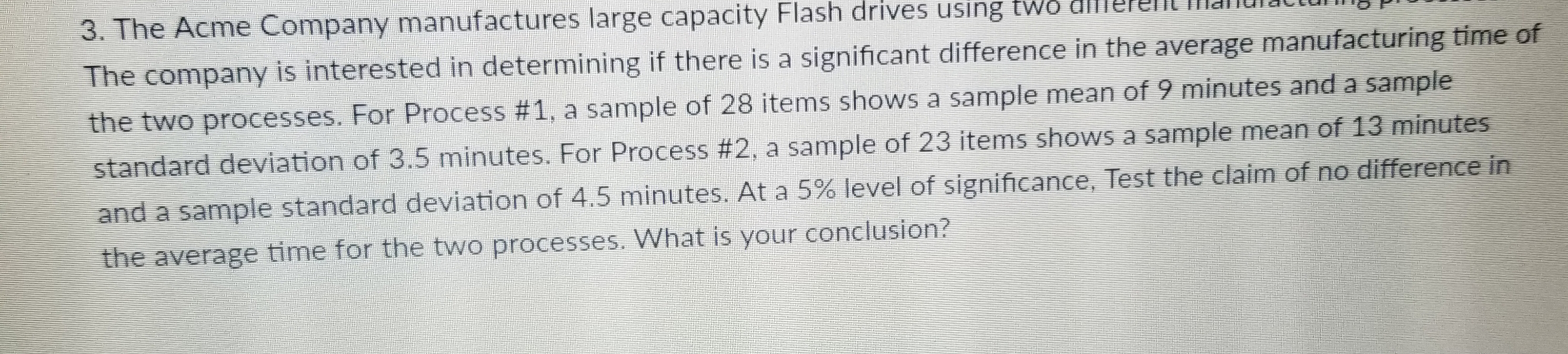# e Acme Company manufactures large capacity Flash drives using tws dlllereThe company is interested in determining if there is a significant difference in the average manufacturing time ofthe two processes. For Process #1, a sample of 28 items shows a sample mean of 9 minutes and a samplestandard deviation of 3.5 minutes. For Process #2, a sample of 23 items shows a sample mean of 13 minutesand a sample standard deviation of 4.5 minutes. At a 5% level of significance, Test the claim of no difference inthe average time for the two processes. What is your conclusion?

Question
54 views

Question #3 i started problem little

P#1 mean =9 SD= 3.5 n=  28

P#2 mean 13 SD= 4.5 n=23

Chpt  10help_outlineImage Transcriptionclosee Acme Company manufactures large capacity Flash drives using tws dlllere The company is interested in determining if there is a significant difference in the average manufacturing time of the two processes. For Process #1, a sample of 28 items shows a sample mean of 9 minutes and a sample standard deviation of 3.5 minutes. For Process #2, a sample of 23 items shows a sample mean of 13 minutes and a sample standard deviation of 4.5 minutes. At a 5% level of significance, Test the claim of no difference in the average time for the two processes. What is your conclusion? fullscreen
check_circle

Step 1

The aim is to check whether there is a difference between the average manufacturing times in the two process. Here the sample sizes are 28 and 23 respectively which are comparatively less and the population standard deviations are unknown. Therefor z test cannot be used here. In this situation t test can be used.

Let μ1 be the true average manufacturing time of the 1st process.

Let μ2 be the true average manufacturing time of the 2nd process.

Let x1 bar and s1 be the sample mean and sample standard deviation manufacturing time of the 1st process.

Let x2 bar and s2 be the sample mean and sample standard deviation manufacturing time of the 2nd process.

Step 2

State the appropriate null and alternative hypotheses:

Null hypothesis:

H0 : μ1 = μ2

That is, the average manufacturing times in the two process is same.

Alternative hypothesis:

H1 : μ1 ≠ μ2

That is, the average manufacturing times in the two process differs from each other.

Test statistic:

The test statistic for t test is given by:

Step 3

Here, n1 = 28, n2 = 23, x1 bar = 9, x2 bar = 13, s1 = 3.5, s2 = 4.5

Therefore the ...

### Want to see the full answer?

See Solution

#### Want to see this answer and more?

Solutions are written by subject experts who are available 24/7. Questions are typically answered within 1 hour.*

See Solution
*Response times may vary by subject and question.
Tagged in

### Statistics